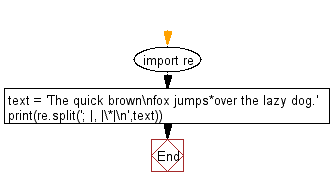﻿ Python Exercises: Split a string with multiple delimiters - w3resource# Python: Split a string with multiple delimiters

## Python Regular Expression: Exercise-47 with Solution

Write a Python program to split a string with multiple delimiters.

Note : A delimiter is a sequence of one or more characters used to specify the boundary between separate, independent regions in plain text or other data streams. An example of a delimiter is the comma character, which acts as a field delimiter in a sequence of comma-separated values.

Sample Solution:-

Python Code:

``````import re
text = 'The quick brown\nfox jumps*over the lazy dog.'
print(re.split('; |, |\*|\n',text))
```
```

Sample Output:

```['The quick brown', 'fox jumps', 'over the lazy dog.']
```

Pictorial Presentation:Flowchart:## Visualize Python code execution:

The following tool visualize what the computer is doing step-by-step as it executes the said program:

Python Code Editor:

Have another way to solve this solution? Contribute your code (and comments) through Disqus.

What is the difficulty level of this exercise?

Test your Python skills with w3resource's quiz

﻿

## Python: Tips of the Day

Merges two or more lists into a list of lists, combining elements from each of the input lists based on their positions

Example:

```def tips_merge(*args, fill_value=None):
max_length = max([len(lst) for lst in args])
result = []
for i in range(max_length):
result.append([
args[k][i] if i < len(args[k])
else fill_value for k in range(len(args))
])
return result
print(tips_merge(['x', 'y'], [1, 2], [True, False]))
print(tips_merge(['x'], [1, 2], [True, False]))
print(tips_merge(['x'], [1, 2], [True, False], fill_value = '_'))
```

Output:

```[['x', 1, True], ['y', 2, False]]
[['x', 1, True], [None, 2, False]]
[['x', 1, True], ['_', 2, False]]
```